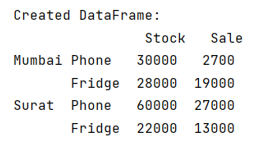# Construct pandas DataFrame from items in nested dictionary

Given a nested dictionary, we have to construct Pandas DataFrame from it items. By Pranit Sharma Last updated : September 23, 2023

Pandas is a special tool that allows us to perform complex manipulations of data effectively and efficiently. Inside pandas, we mostly deal with a dataset in the form of DataFrame. DataFrames are 2-dimensional data structures in pandas. DataFrames consist of rows, columns, and data.

## Constructing pandas DataFrame from items in nested dictionary

To create pandas DataFrame from items in a nested dictionary, we will use the following syntax:

```pd.DataFrame.from_dict({(i,j): d[i][j] for i in d.keys() for j in d[i].keys()},orient='index')
```

If we have a very huge nested dictionary, this method allows us to extract keys inside keys and fetch their values to create columns and rows of the DataFrame.

Note

To work with pandas, we need to import pandas package first, below is the syntax:

```import pandas as pd
```

Let us understand with the help of an example,

## Python program to construct pandas DataFrame from items in nested dictionary

```# Importing pandas package
import pandas as pd

# Creating a nested dictionary
d = {
'Mumbai':{
'Phone':{'Stock':30000,'Sale':2700},
'Fridge':{'Stock':28000,'Sale':19000}
},
'Surat':{
'Phone':{'Stock':60000,'Sale':27000},
'Fridge':{'Stock':22000,'Sale':13000}
}
}

# Creating a DataFrame
df = pd.DataFrame.from_dict({(i,j): d[i][j] for i in d.keys() for j in d[i].keys()},orient='index')

# Display DataFrame
print("Created DataFrame:\n",df)
```

### Output

The output of the above program is: